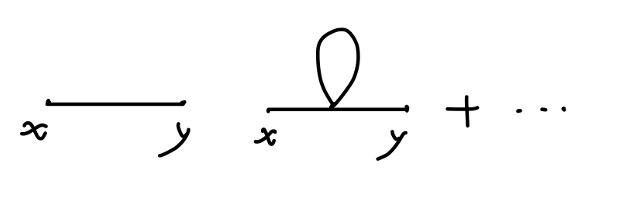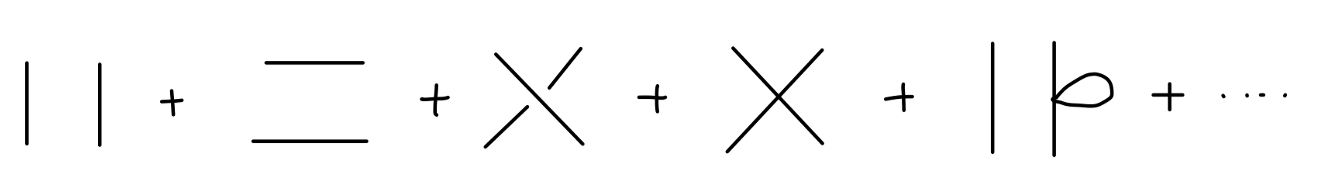## 费曼图与费曼规则

### 费曼图

$\langle 0|T\{\phi(x)\phi(y)\}|0\rangle = D_F(x-y) \tag{1}$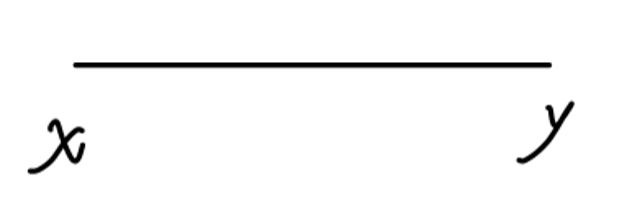Fig1：自由两点关联函数

\begin{aligned} \langle 0|T\{\phi_1\phi_2\phi_3\phi_4\}|0\rangle &= D_F(x_1-x_2)D_F(x_3-x_4)\\ & + D_F(x_1-x_3)D_F(x_2-x_4) + D_F(x_1-x_4)D_F(x_2-x_3)\\ \end{aligned}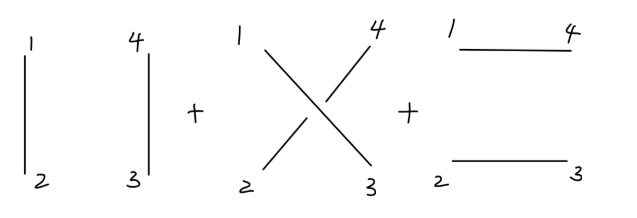Fig2：自由四点关联函数

$\langle \Omega|T\{\phi(x)\phi(y)\}|\Omega\rangle =\frac{\langle 0|T\{\phi_I(x)\phi_I(y)\exp(-i\int_{-\infty}^{\infty}dtH_I(t))|0\rangle}{\langle 0|T\{\exp(-i\int_{-\infty}^{\infty}dtH_I(t))\}|0\rangle} \tag{2}$

$\langle 0|T\{\phi(x)\phi(y) + \phi(x)\phi(y) [ -i\int dtH_I(t) ] + \cdots\}|0\rangle \tag{3}$

$H_I = \int \frac{\lambda}{4!}\phi^4(z) d^3z \tag{4}$

$(3)$ 中的零阶项就是自由两点关联函数。一阶项为：

\begin{aligned} &\langle 0| T\{\phi(x)\phi(y) (-i)\int dt \int \frac{\lambda}{4!}\phi^4(z) d^3z \} |0\rangle\\ =& \langle 0| T\{\phi(x)\phi(y) (-i)\int \frac{\lambda}{4!}\phi^4(z) d^4z \} |0\rangle\\ =& \langle 0| T\{\phi(x)\phi(y) (\frac{-i\lambda}{4!})\int \phi(z)\phi(z)\phi(z)\phi(z) d^4z \} |0\rangle\\ \end{aligned}\tag{5}

• $\phi(x),\phi(y)$ 缩并，$\phi(z)$ 间两两缩并。例如：

\begin{aligned} &\langle 0| \phi^{\bullet}(x)\phi^{\bullet}(y)(\frac{-i\lambda}{4!})\int \phi^{\bullet\bullet}(z)\phi^{\bullet\bullet}(z)\phi^{(3\bullet)}(z)\phi^{(3\bullet)}(z) d^4z \} |0\rangle\\ =& \frac{-i\lambda}{4!} D_F(x-y) \int d^4z D_F(z-z)D_F(z-z) \end{aligned}

$C_{2}^{2}\frac{C_{2}^{4}}{A^{2}_{2}} = 3$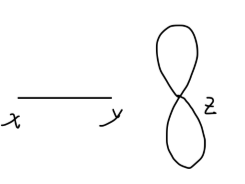Fig3：$\phi^4$ 两点关联函数一阶项：第一种缩并方式

• $\phi(x),\phi(y)$ 分别与 $\phi(z)$ 缩并，剩下的两个 $\phi(z)$ 缩并。例如：

\begin{aligned} &\langle 0| \phi^{\bullet}(x)\phi^{\bullet\bullet}(y)(\frac{-i\lambda}{4!})\int \phi^{\bullet}(z)\phi^{\bullet\bullet}(z)\phi^{(3\bullet)}(z)\phi^{(3\bullet)}(z) d^4z \} |0\rangle\\ =& \frac{-i\lambda}{4!} \int d^4z D_F(x-z)D_F(y-z)D_F(z-z) \end{aligned}

$C_{1}^{4}C_{1}^{3} = 12$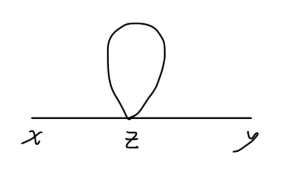Fig4：$\phi^4$ 两点关联函数一阶项：第二种缩并方式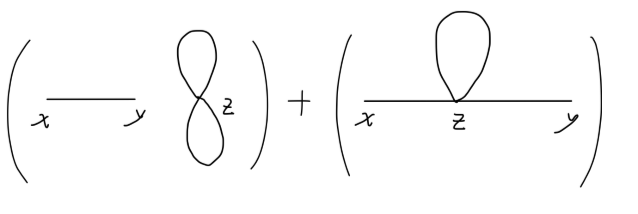Fig5：$\phi^4$ 两点关联函数一阶项

$\langle 0| \phi^{\bullet}(x)\phi^{\star}(y) \frac{1}{3!}(\frac{-i\lambda}{4!})^3 \int d^4z \phi^{\bullet}\phi^{\bullet\bullet}\phi^{\bullet\bullet} \phi^{(3\bullet)} \int d^4w \phi^{(3\bullet)} \phi^{\star}\phi^{\star\star}\phi^{(3\star)} \int d^4u \phi^{\star\star}\phi^{\star\star}\phi^{(4\star)}\phi^{(4\star)}$

$A_{3}^{3}C^{4}_{1}C^{3}_{2}C^{4}_{1}C^{3}_{1}C^{4}_{2}C^{2}_{1} = 10368$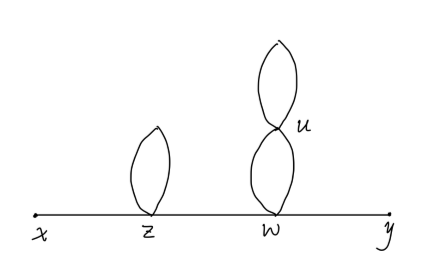Fig6：$\phi^4$ 两点关联函数三阶项的一种贡献

### 费曼规则

• 顶点 vertex
• 传播子 propagator
• 外部点 external point

Fig6 的值中出现的元素为：

• 积分 $\int d^4z$
• 耦合常数 $\lambda/4!$
• 传播子 $D_F(x-y)$
• 对称因子 symmetry factor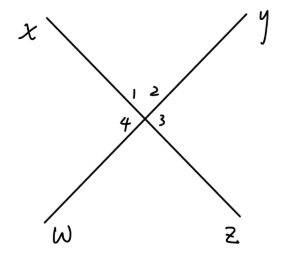Fig7：对称度例1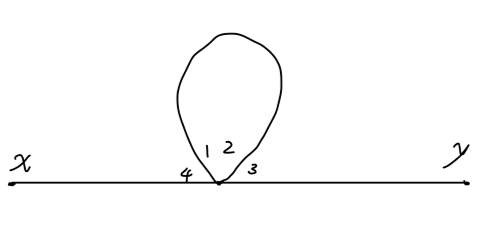Fig8：对称度例2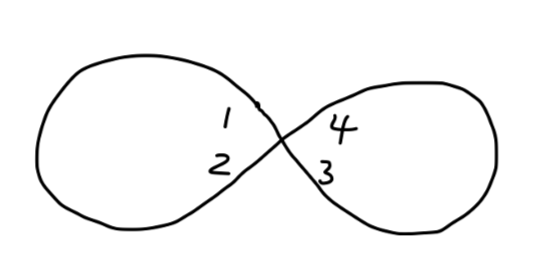Fig9：对称度例3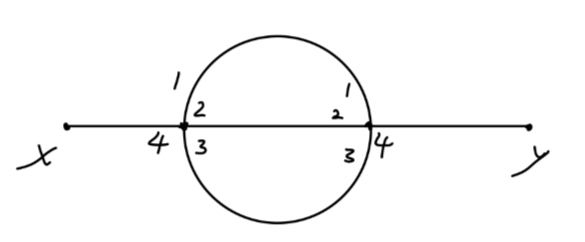Fig10：对称度例4

$4!\times 4!\times 2! = 1152$

$1152/6 = 192$

$(4!)^nn!$

$(4!)^nn!/S$

$(4!)^nn! \sum_i S_{i}^{-1} \tag{6}$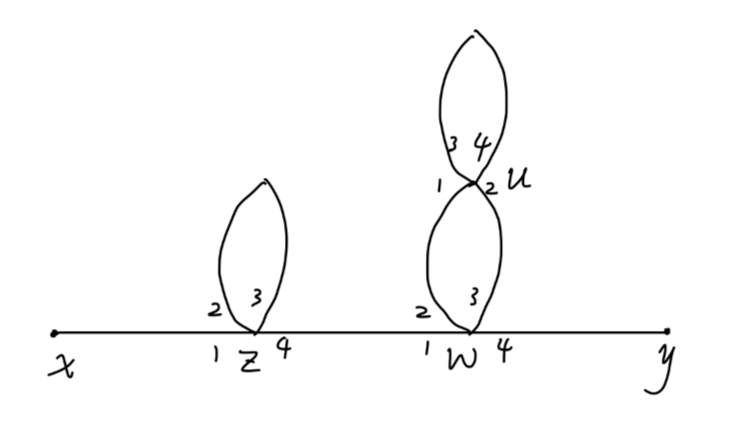Fig11：对称度例5

• 交换 $z$ 顶点线 $2,3$$S_1 = 2$
• 交换 $w$ 顶点线 $2,3$$S_2 = 2$
• 交换 $u$ 顶点线 $2,3$$S_3 = 2$

$S = S_1S_2S_3 = 8$

$\frac{(4!)^3\times 3!}{8} = 10368$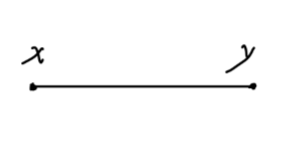Fig12：传播子

$D_F(x-y)$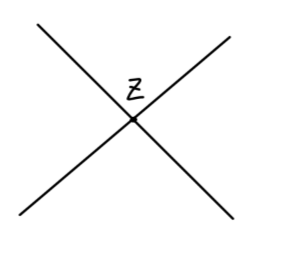Fig13：顶点

$\phi^{4}$ 理论中，顶点应当对应于：

$\int \frac{-i\lambda}{4!}d^4z \tag{7}$

$\int -i\lambda d^4z \tag{8}$

$\frac{n!}{S}$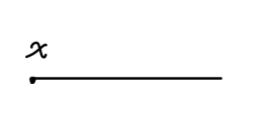Fig14：外部点

• 传播子值为：

$D_F(x-y)$

• 顶点值为：

$\int -i\lambda d^4z$

• 外部点值为：

$1$

• 除以对称因子 $S$

$D_F(x-y) = \int \frac{d^4p}{(2\pi)^4} \frac{i}{p^2 -m^2 + i\epsilon} e^{-ip(x-y)}$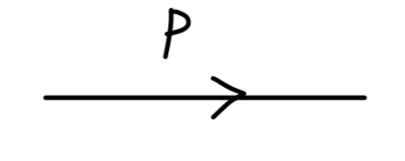Fig15：传播子

$\frac{i}{p^2 -m^2 + i\epsilon}$

$e^{-ip(x-y)} = e^{-ipx} e^{ipy}$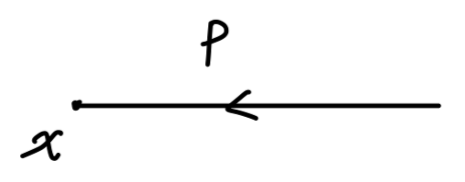Fig16：外部点

$e^{-ipx}$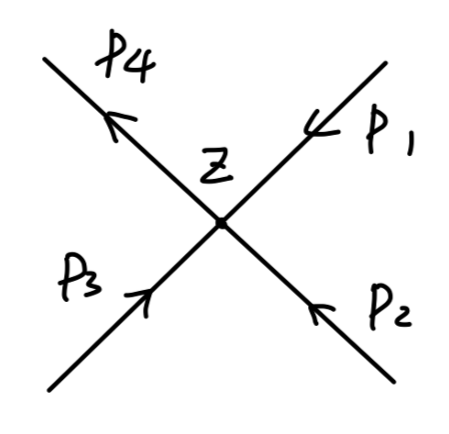Fig17：顶点

\begin{aligned} & -i\lambda \int d^4z e^{-ip_1z}e^{-ip_2z}e^{-ip_3z}e^{+ip_4z} \\ =& -i\lambda (2\pi)^4 \delta(-p_1-p_2-p_3+p_4)\\ \end{aligned}

$\phi^4$ 理论动量空间中的费曼规则

• 传播子$\frac{i}{p^2 - m^2 + i\epsilon}$

• 顶点$-i\lambda$

• 外部点$e^{-ip\cdot x}$

• 对每个顶点应用四动量守恒。

• 对未确定的动量积分

$\int \frac{d^4p}{(2\pi)^4}$

• 除以对称因子 $S$

### 两点关联函数的计算

• 连通图 connected diagram：图中任意两点都是连通的。
• 非连通图 disconnected diagram：存在两点不相连通。$\phi^4$ 理论两点关联函数的计算中，一个费曼图总有两个外部点。那么一般情况下，一个典型的两点关联函数的费曼图为：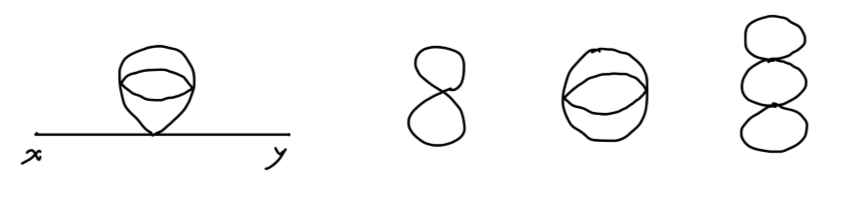Fig18：两点关联函数的费曼图

$(value\ of\ connected\ piece)\cdot \prod_{i}\frac{1}{n_i!}(V_i)^{n_i}$

\begin{aligned} &\sum_{connected \ pieces} \sum_{all\ \{n_i\}} \left\{\begin{aligned} &\qquad value\ of\\ &connected\ piece\\ \end{aligned}\right\} \times \prod_{i}\frac{1}{n_i!}(V_i)^{n_i}\\ &= (\sum connected)\times \sum_{all\ \{n_i\}} \prod_{i}\frac{1}{n_i!}(V_i)^{n_i}\\ &= (\sum connected)\times \prod_{i} \sum_{n_i}\frac{1}{n_i!}(V_i)^{n_i}\\ &= (\sum connected)\times \prod_{i} \exp(V_i) \\ &= (\sum connected)\times \exp(\sum_{i} V_i) \\ \end{aligned}

$\langle 0|T\{\phi(x)\phi(y)\exp[-i\int_{-\infty}^{\infty}dtH_I(t)]\}|0\rangle$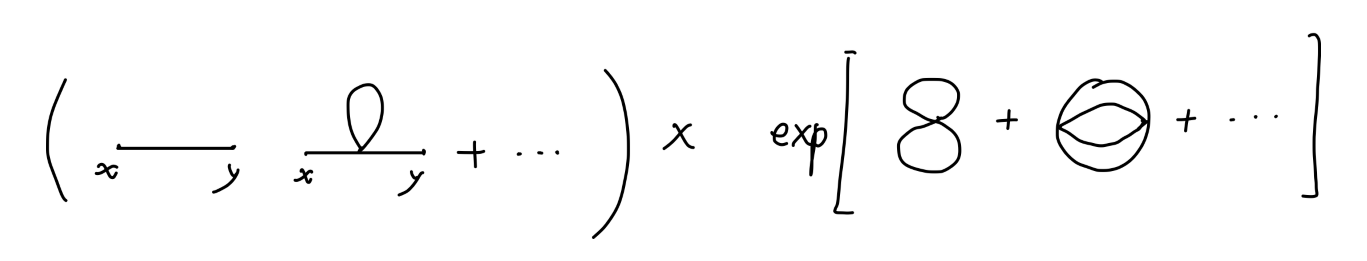$\langle 0|T\{\exp[-i\int_{-\infty}^{\infty}dtH_I(t)]\}|0\rangle$

$\exp(\sum_i V_i)$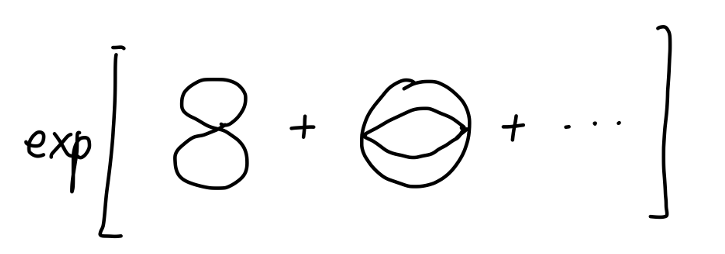$\langle\Omega|T\{\phi(x)\phi(y)\}|\Omega\rangle = \sum connected \tag{9}$